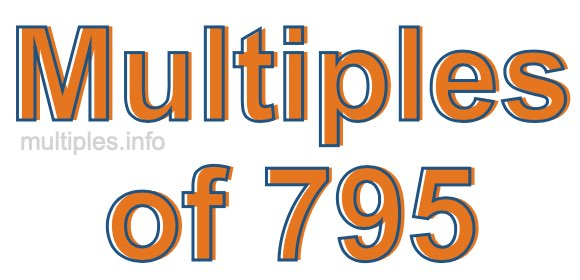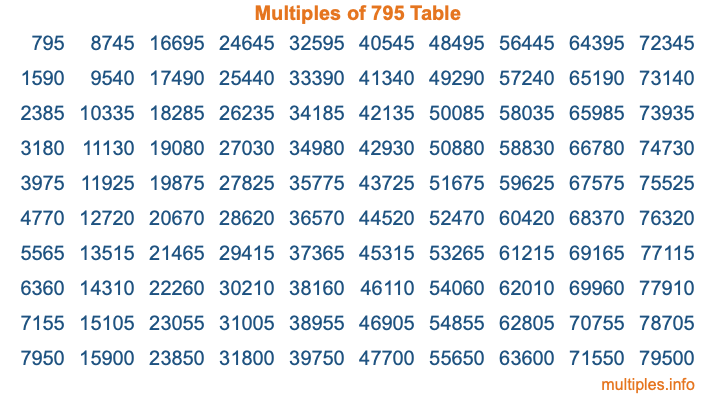Multiples of 795Welcome to the Multiples of 795 page. Here we will first teach you everything you will ever need to know about the multiples of 795, and then give you a study guide summary of everything we taught you to make sure you remember it all. Use this page to look up facts and learn information about the multiples of 795. This page will make you a multiples of seven hundred ninety-five expert!

Definition of Multiples of 795
Multiples of 795 are all the numbers that when divided by 795 equal an integer. Each of the multiples of 795 are called a multiple. A multiple of 795 is created by multiplying 795 by an integer.

Therefore, to create a list of multiples of 795, you start with 1 multiplied by 795, then 2 multiplied by 795, then 3 multiplied by 795, and so on for as long as you want. Thus, the list of the first five multiples of 795 is 795, 1590, 2385, 3180, and 3975. To see a larger list of multiples of 795, see the printable image of Multiples of 795 further down on this page. We also have a category where you can choose any nth multiple of 795.

Multiples of 795 Checker
The Multiples of 795 Checker below checks to see if any number of your choice is a multiple of 795. In other words, it checks to see if there is any number (integer) that when multiplied by 795 will equal your number. To do that, we divide your number by 795. If the the quotient is an integer, then your number is a multiple of 795.

Is  a multiple of 795?

Least Common Multiple of 795 and ...
A Least Common Multiple (LCM) is the lowest multiple that two or more numbers have in common. This is also called the smallest common multiple or lowest common multiple and is useful to know when you are adding our subtracting fractions. Enter one or more numbers below (795 is already entered) to find the LCM.

Check out our LCM Calculator if you need more details about the Least Common Multiple or if you need the LCM for different numbers for adding and subtraction fractions.

nth Multiple of 795
As we stated above, 795 is the first multiple of 795, 1590 is the second multiple of 795, 2385 is the third multiple of 795, and so on. Enter a number below to find the nth multiple of 795.

th multiple of 795

Multiples of 795 vs Factors of 795
795 is a multiple of 795 and a factor of 795, but that is where the similarities end. All postive multiples of 795 are 795 or greater than 795. All positive factors of 795 are 795 or less than 795.

Below is the beginning list of multiples of 795 and the factors of 795 so you can compare:

Multiples of 795: 795, 1590, 2385, 3180, 3975, etc.

Factors of 795: 1, 3, 5, 15, 53, 159, 265, 795

As you can see, the multiples of 795 are all the numbers that you can divide by 795 to get a whole number. The factors of 795, on the other hand, are all the whole numbers that you can multiply by another whole number to get 795.

It's also interesting to note that if a number (x) is a factor of 795, then 795 will also be a multiple of that number (x).

Multiples of 795 vs Divisors of 795
The divisors of 795 are all the integers that 795 can be divided by evenly. Below is a list of the divisors of 795.

Divisors of 795: 1, 3, 5, 15, 53, 159, 265, 795

The interesting thing to note here is that if you take any multiple of 795 and divide it by a divisor of 795, you will see that the quotient is an integer.

Multiples of 795 Table
Below is an image of the first 100 multiples of 795 in a table. The table is in chronological order, column by column. The first column has the first ten multiples of 795, the second column has the next ten multiples of 795, and so on.The Multiples of 795 Table is also referred to as the 795 Times Table or Times Table of 795. You are welcome to print out our table for your studies.

Negative Multiples of 795
Although not often discussed or needed in math, it is worth mentioning that you can make a list of negative multiples of 795 by multiplying 795 by -1, then by -2, then by -3, and so on, to get the following list of negative multiples of 795:

-795, -1590, -2385, -3180, -3975, etc.

Multiples of 795 Summary
Below is a summary of important Multiples of 795 facts that we have discussed on this page. To retain the knowledge on this page, we recommend that you read through the summary and explain to yourself or a study partner why they hold true.

There are an infinite number of multiples of 795.

A multiple of 795 divided by 795 will equal a whole number.

795 divided by a factor of 795 equals a divisor of 795.

The nth multiple of 795 is n times 795.

The largest factor of 795 is equal to the first positive multiple of 795.

795 is a multiple of every factor of 795.

795 is a multiple of 795.

A multiple of 795 divided by a divisor of 795 equals an integer.

795 divided by a divisor of 795 equals a factor of 795.

Any integer times 795 will equal a multiple of 795.

Multiples of a Number
Here you can get the multiples of another number, all with the same attention to detail as we did for multiples of 795 on this page.

Multiples of
Multiples of 796
Did you find our page about multiples of seven hundred ninety-five educational? Do you want more knowledge? Check out the multiples of the next number on our list!